CBSE Class 10 Sample Paper for 2020 Boards - Maths Standard

Class 10
Solutions of Sample Papers for Class 10 Boards

## Solve the following system of equations: 21/x + 47/y = 110 47/x + 21/x = 162, x, y ≠ 0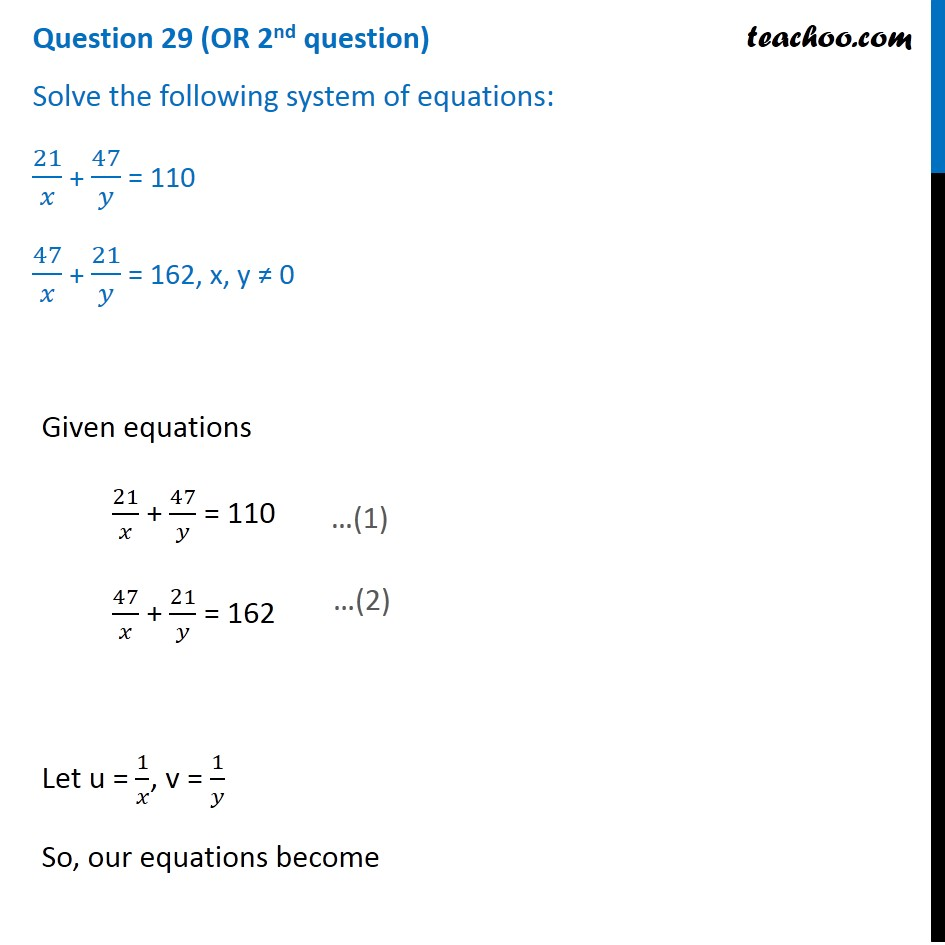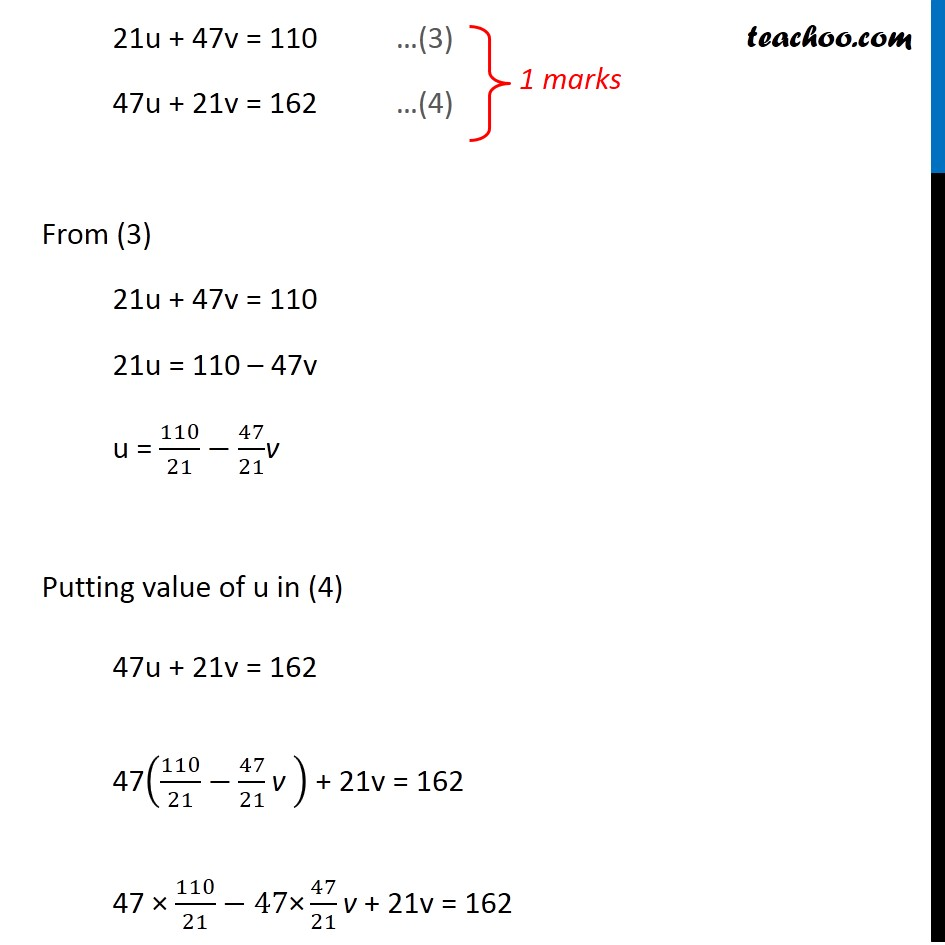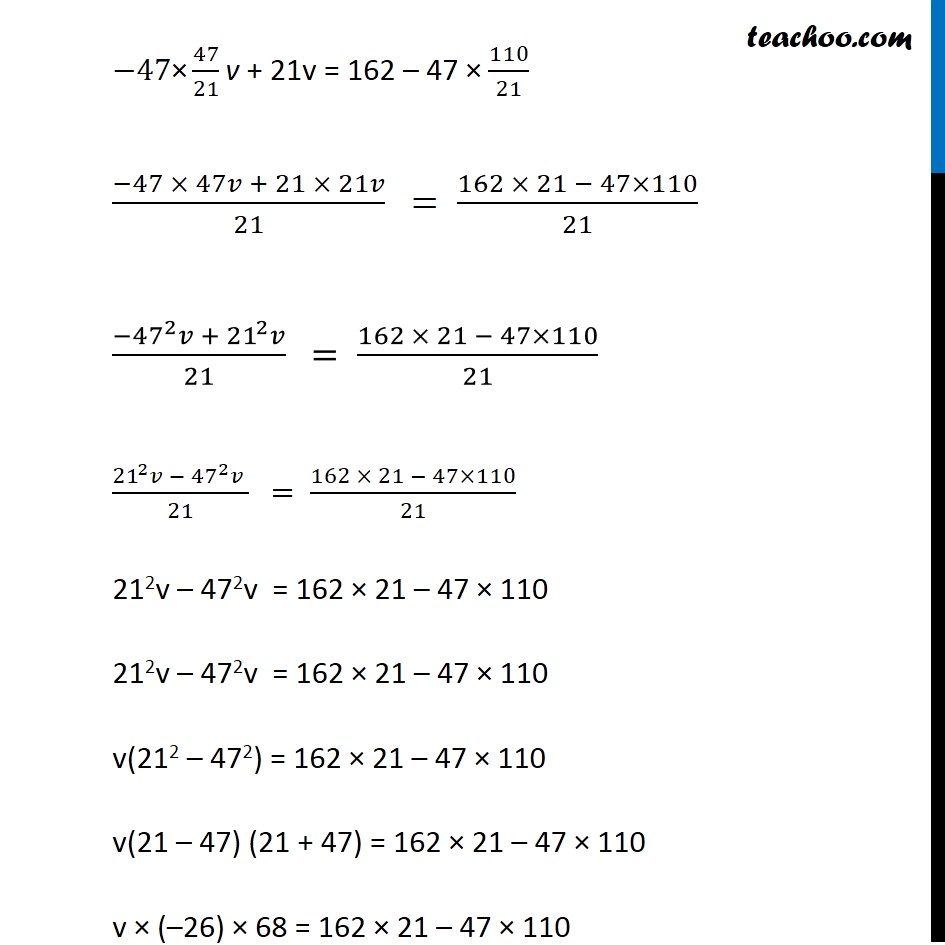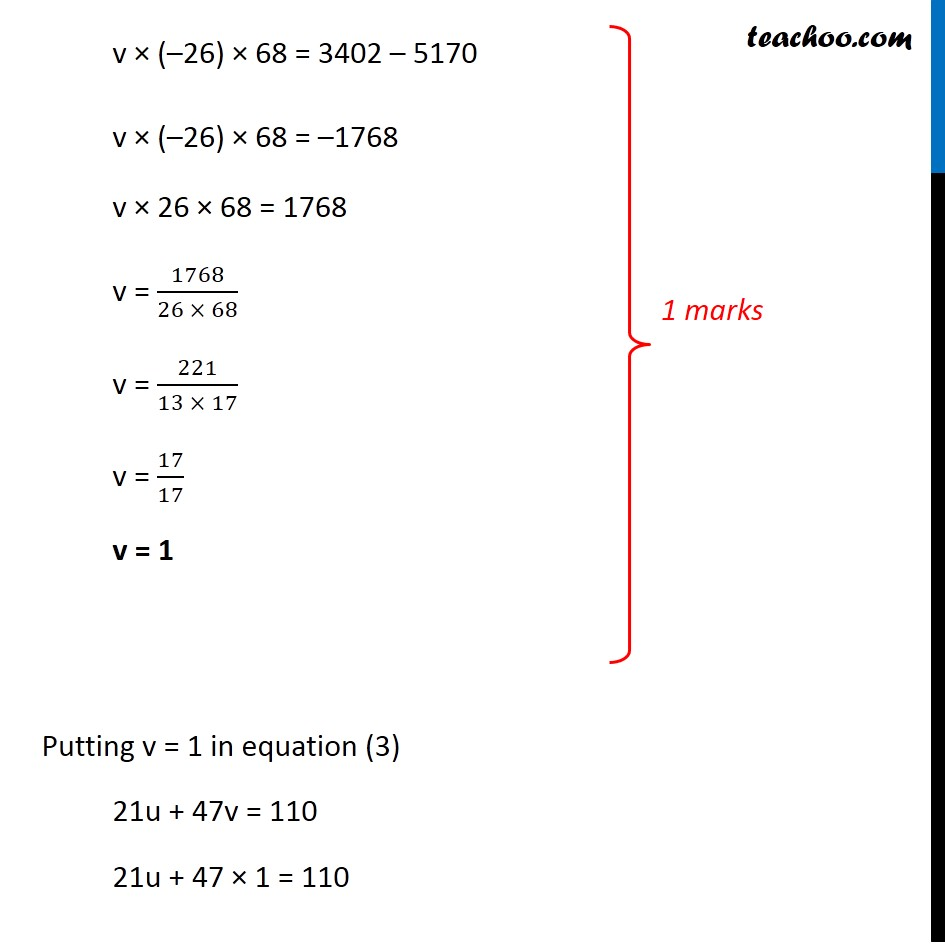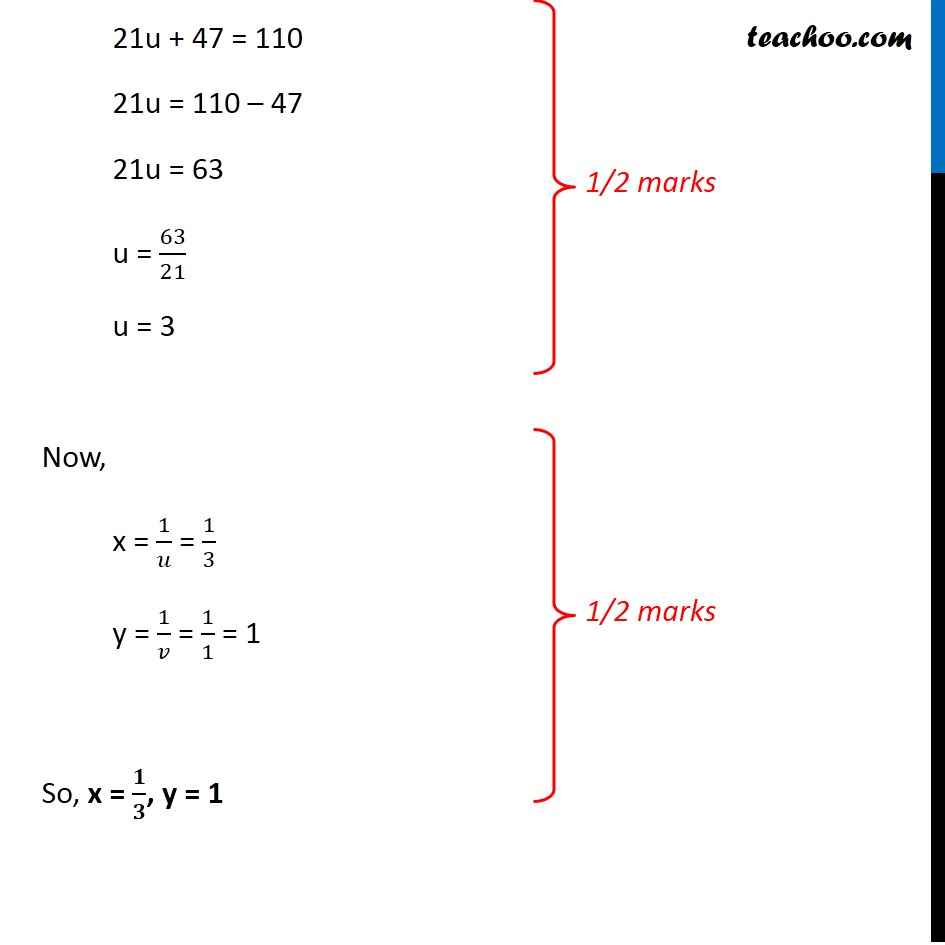Note : This is similar to Example 17 of NCERT – Chapter 3 Class 10

### Transcript

Question 29 (OR 2nd question) Solve the following system of equations: 21/𝑥 + 47/𝑦 = 110 47/𝑥 + 21/𝑦 = 162, x, y ≠ 0 Given equations 21/𝑥 + 47/𝑦 = 110 47/𝑥 + 21/𝑦 = 162 Let u = 1/𝑥, v = 1/𝑦 So, our equations become 21u + 47v = 110 …(3) 47u + 21v = 162 …(4) From (3) 21u + 47v = 110 21u = 110 – 47v u = 110/21−47/21v Putting value of u in (4) 47u + 21v = 162 47(110/21−47/21 "v " ) + 21v = 162 47 × 110/21−47"×" 47/21 "v "+ 21v = 162 −47"×" 47/21 "v "+ 21v = 162 – 47 × 110/21 (−47 × 47𝑣 + 21 × 21𝑣)/21 " "= (162 × 21 − 47×110)/21 (−〖47〗^2 𝑣 + 〖21〗^2 𝑣)/21 " "= (162 × 21 − 47×110)/21 (〖21〗^2 𝑣 − 〖47〗^2 𝑣 )/21 " "= (162 × 21 − 47×110)/21 212v – 472v = 162 × 21 – 47 × 110 212v – 472v = 162 × 21 – 47 × 110 v(212 – 472) = 162 × 21 – 47 × 110 v(21 – 47) (21 + 47) = 162 × 21 – 47 × 110 v × (–26) × 68 = 162 × 21 – 47 × 110 v × (–26) × 68 = 3402 – 5170 v × (–26) × 68 = –1768 v × 26 × 68 = 1768 v = 1768/(26 × 68) v = 221/(13 × 17) v = 17/17 v = 1 Putting v = 1 in equation (3) 21u + 47v = 110 21u + 47 × 1 = 110 21u + 47 = 110 21u = 110 – 47 21u = 63 u = 63/21 u = 3 Now, x = 1/𝑢 = 1/3 y = 1/𝑣 = 1/1 = 1 So, x = 𝟏/𝟑, y = 1